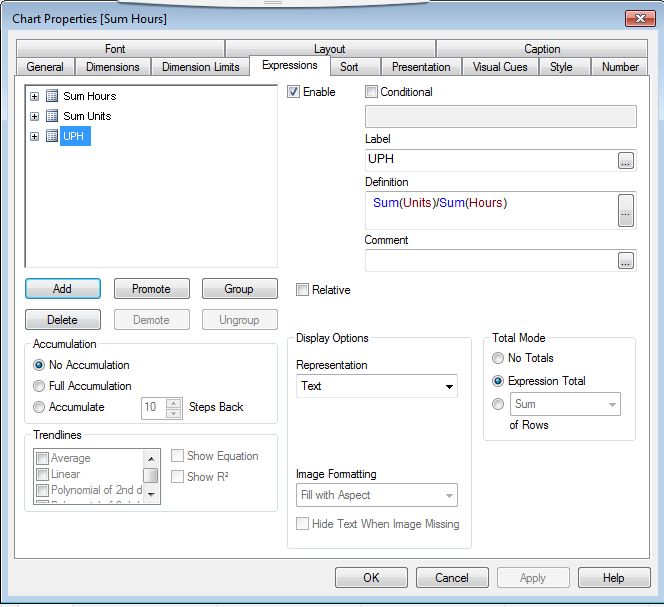# QlikView App Development

Discussion Board for collaboration related to QlikView App Development.

Highlighted
New Contributor II

## Greater Than

Hello,

I'm trying to get this chart to show all records that has (<=40 Hours)Tags (4)
5 Replies
Contributor III

## Re: Greater Than

I am assuming Hours is your dimension in the chart.

Please change the dimension like below.

if(Hours<=40,Hours)

Then Check on the Suppress when value is null

Esteemed Contributor III

## Re: Greater Than

Or may be try like

= Aggr(IF(Hours<=40, Name), Name)

Suppress when value is null for this dimension.MVP

## Re: Greater Than

Is Hour a field or a calculation check? You can try this as a set analysis like this

If it is a calculation

Sum({<Name = {"=Sum(Hours) <= 40"}>}Units)

If it is a field

Sum({<Hours =  {"<=40"}>}Units)

New Contributor II

## Re: Greater Than

Hello All,

Thanks for helping I've tried all the formulas, and I still haven't figured it out. The images below are the current dimensions, and fields selected. Oh and I forgot to say..... I'm a noob, I've only been working with Qlik for a couple of weeks.

Thanks,MVP

## Re: Greater Than

May be use Name as dimension and this expressions

Sum({<Hours =  {"<=40"}>}Units)

Sum({<Hours =  {"<=40"}>}Hours)

Sum({<Hours =  {"<=40"}>}Units)/Sum({<Hours =  {"<=40"}>}Hours)